SNVS359E May   2005  – December 2014

PRODUCTION DATA.

1. Features
2. Applications
3. Description
4. Revision History
5. Pin Configuration and Functions
6. Specifications
7. Detailed Description
1. 7.1 Overview
2. 7.2 Functional Block Diagram
3. 7.3 Feature Description
4. 7.4 Device Functional Modes
8. Application and Implementation
1. 8.1 Application Information
2. 8.2 Typical Application
1. 8.2.1 Design Requirements
2. 8.2.2 Detailed Design Procedure
3. 8.2.3 Application Curves
9. Power Supply Recommendations
10. 10Layout
11. 11Device and Documentation Support
12. 12Mechanical, Packaging, and Orderable Information

• DGK|8
• P|8
• DGK|8

## 8 Application and Implementation

NOTE

Information in the following applications sections is not part of the TI component specification, and TI does not warrant its accuracy or completeness. TI’s customers are responsible for determining suitability of components for their purposes. Customers should validate and test their design implementation to confirm system functionality.

### 8.1 Application Information

#### 8.1.1 Startup Circuit

Referring to Figure 13, the input capacitor CVIN is trickle charged through the start-up resistor Rstart, when the rectified ac input voltage HV is applied. The VIN current consumed by the LM5021 is only 18 µA (nominal) while the capacitor CVIN is initially charged to the start-up threshold. When the input voltage, VIN reaches the upper VIN UVLO threshold of 20 V, the internal VCC linear regulator is enabled. The VCC regulator will remain on until VIN falls to the lower UVLO threshold of 7.25 V (12.5 V hysteresis). When the VCC regulator is turned on, the external capacitor at the VCC pin begins to charge. The PWM controller, soft-start circuit and gate driver are enabled when the VCC voltage reaches the VCC UVLO upper threshold of 7 V. The VCC UVLO has 1.2 V hysteresis between the upper and lower thresholds to avoid chattering during transients on the VCC pin. When the VCC UVLO enables the switching power supply, energy is transferred from the primary to the secondary transformer winding(s). A bias winding, shown in Figure 13, delivers power to the VIN pin to sustain the VCC regulator. The voltage supplied should be from 11 V (VCC regulated voltage maximum plus VCC regulator dropout voltage) to 30 V (maximum operating VIN voltage). The bias winding should always be connected to the VIN pin as shown in Figure 13. Do not connect the bias winding to the VCC pin. The start-up sequence is completed and normal operation begins when the voltage from the bias winding is sufficient to maintain VCC level greater than the VCC UVLO threshold (5.8 V typical).

The LM5021 is designed for ultra-low start-up current into the VIN pin. To achieve this very low start-up current, the VCC regulator of the LM5021 is unique as compared to the VCC regulator used in other controllers of the LM5xxx family. The LM5021 is designed specifically for applications with the bias winding connected to the VIN pin as shown in Figure 13.

NOTE

It is not recommended that the bias winding be connected to the VCC pin of the LM5021. Doing so can cause the device to operate incorrectly or not at all.

The size of the start-up resistor Rstart not only affects power supply start-up time, but also power supply efficiency since the resistor dissipates power in normal operation. The ultra low start-up current of the LM5021 allows a large value Rstart resistor (up to 3 MΩ) for improved efficiency with reasonable start-up time.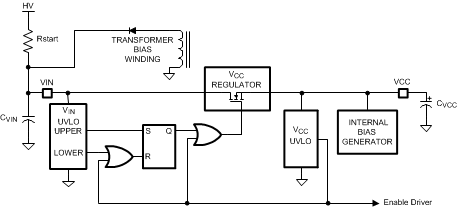Figure 13. Start-Up Circuit Block Diagram

#### 8.1.2 Relationship Between Input Capacitor CIN and VCC Capacitor CVCC

The internal VCC linear regulator is enabled when VIN reaches 20 V. The drop in VIN due to charge transfer from CVIN to CVCC after the regulator is enabled can be calculated from the following equations where VIN' is the voltage on CVIN immediately after the VCC regulator charges CVCC.

Equation 5. ΔVIN x CVIN = ΔVCC x CVCC
Equation 6. (20 V – VIN') CVIN = 8.5V CVCC
Equation 7.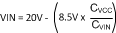Assuming CVIN value as 10 µF, and CVCC of 1µF, then the drop in VIN will be 0.85 V, or the VIN value drops to 19.15 V. The value of the VCC capacitor can be small (less than 1 uF) as it supplies only transient gate drive current of a short duration. The CVIN capacitor must be sized to supply the gate drive current and the quiescent current of LM5021, until the transformer bias winding delivers sufficient voltage to VIN to sustain the VCC voltage.

The CVIN capacitor value can be calculated from the operating VCC load current after its output voltage reaches the VCC UVLO threshold. For example, if the LM5021 is driving an external MOSFET with total gate charge (Qg) of 25nC, the average gate drive current is Qg x Fsw, where Fsw is the switching frequency. Assuming a switching frequency of 150KHz, the average gate drive current is 3.75 mA. Since the IC consumes approximately 2.5 mA operating current in addition to the gate current, the total current drawn from CVIN capacitor is the operating current plus the gate charge current, or 6.25 mA. The CVIN capacitor must supply this current for a brief time until the transformer bias winding takes over. The CVIN voltage must not fall below 8.5 V during the start-up sequence or the cycle will be restarted. The maximum allowable start-up time can be calculated using the value of CVIN, the change in voltage allow at VIN (19.15 V – 8.5 V) and the VCC regulator current (6.25 mA). Tmax, the maximum time allowed to energize the bias winding is:

Equation 8.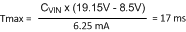If the calculated value of Tmax is too small, the value of Cin should be increased further to allow more time before the transformer bias winding takes over and delivers the operating current to the VCC regulator. Increasing CVIN will increase the time from the application of the rectified ac (HV in Figure 13) to the time when VIN reaches the 20 V start threshold. The initial charging time of CVIN is:

Equation 9.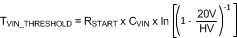### 8.2 Typical ApplicationFigure 14. Typical Application Circuit

#### 8.2.1 Design Requirements

DESIGN PARAMETER VALUE
Input voltage range 85 Vac - 130 Vac
Output voltage 24 Vdc or 8 Vdc (programmable)
Output current 1.45 Adc at 24 Vdc
Switching frequency 145 kHz
Maximum duty cycle 50%
Isolation level 4 kV
Footprint 68 mm × 34 mm

#### 8.2.2.1 Primary Bulk Capacitance

The primary side bulk cap, C4, is selected based on the power level and the desired minimum bulk voltage level. The bulk capacitor value can be calculated as:

Equation 10.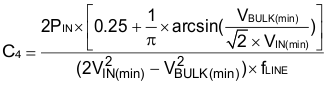where

• PIN is the maximum input power. Input power is the maximum output power divided target efficiency.
• VIN(min) is the minimum AC input voltage RMS value.
• VBULK(min) is the target minimum bulk voltage.
• fLINE is the line frequency.

Based on the equation, to achieve 70-V minimum bulk voltage, the bulk capacitor should be larger than 72 µF and 82 µF was chosen in the design.

#### 8.2.2.2 Transformer

The transformer design starts with selecting a suitable switching frequency. Generally, the switching frequency selection is based on the tradeoff between the converter size and efficiency. Higher switching frequency results in smaller transformer size, but the switching losses will increase, potentially impacting efficiency. Sometimes, the switching frequency is selected to avoid certain frequencies or harmonics that could interfere with those used for communication. The frequency selection is beyond the scope of this datasheet.

EMI regulations place limits on EMI noise at 150 kHz and higher. For this design, 145 kHz is selected for the switching frequency to minimize transformer size while keeping the switching frequency below the EMI regulation band.

The transformer turns ratio can be selected based on the desired MOSFET voltage rating and diode voltage rating. Since the maximum input voltage is 130 V AC, the peak bulk voltage can be calculated as:

Equation 11. VBULK(max) = √2 × VIN(max) = 184 V

To take advantage of the low Rdson of lower voltage MOSFETs, a target device rating of 400 V is selected. Considering the design margin and extra voltage ringing on the MOSFET drain, the reflected output voltage should be less than 50 V. The transformer primary to secondary (nPS) turns ratio can be selected as:

Equation 12.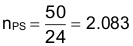The output rectifier diode (D4) voltage stress is also affected by the turns ratio. The stress applied to the diode is the output voltage plus the reflected input voltage. The voltage stress on the diode can be calculated as:

Equation 13.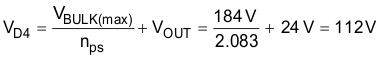Considering the ringing voltage spikes always present in a switching power supply and allowing for voltage derating (normally 80% derating is used), the diode voltage rating should be higher than 150 V.

The transformer inductance selection is based on the requirement for this converter to remain in discontinuous conduction (DCM). Selecting a larger inductance would allow the converter operate in continuous conduction (CCM). CCM operation tends to increase the transformer size. The primary inductance (Lm) can be calculated as:

Equation 14.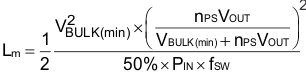In this equation, fsw is the 145-kHz switching frequency. Therefore, the transformer inductance should be selected as 85 µH.

The auxiliary winding provides the power for LM5021 during normal operation. The auxiliary winding voltage is the output voltage reflected to the primary side. A higher reflected voltage allows the IC to quickly get energy from the transformer during startup and makes starting a heavy or highly capacitive load easier. However, a high auxiliary reflected voltage makes the IC consume more power, reducing efficiency and increasing standby power consumption. Therefore, a tradeoff is required. In this design, the auxiliary winding voltage is selected to ensure that there is enough voltage available to ensure the controller will operate when the output voltage is programmed to the lower 8-V setting. Therefore, the auxiliary winding to the output winding turns ratio is selected as:

Equation 15.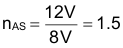#### 8.2.2.3 Main Switch FET and Output Rectifier

Based on calculated inductor value and the switching frequency, the current stress of the MOSFET (Q4) and diode (D4) can be calculated.

The peak current of Q4 can be calculated as:

Equation 16.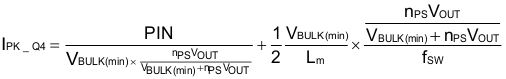The peak current is 2.55 A.

The peak current in D4 is the peak current in Q4 reflected to the secondary side:

Equation 17. ID4 = NPS × IQ4 = 5.3 A

The RMS current in Q4 can be calculated as:

Equation 18.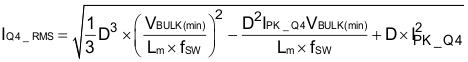Here D is the Q4 on time duty cycle at minimum bulk voltage and it can be calculated as:

Equation 19.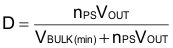The RMS current in Q4 is 0.97 A. Therefore, STP11NK40ZFP is selected.

The average current in D4 is the output current 1.45 A. With a 150-V reverse voltage rating and a 20-A average current rating, 20CTQ150 is selected.

The output capacitor is selected based on the output voltage ripple requirement. In this design, 0.1% voltage ripple is assumed. Based on the 0.1% ripple requirement, the capacitor value can be selected based on:

Equation 20.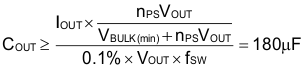Considering the tolerance and temperature effect, together with the ripple current rating of the capacitors, the output capacitor is selected as two 220 uF units in parallel.

#### 8.2.2.4 Timing Resistor

The switching frequency is set by R17. From Equation 2:

Equation 21.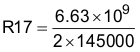Choose R17 as 22.1 k as a common resistor close to the computed value.

#### 8.2.2.5 Soft-Start Time

The soft start time is set by C14. This determines the rate of increase of converter primary peak current at startup. Set a time that is long enough so that the feedback lop can compensate for the transition from open loop during soft start to being closed loop as it takes over from soft start. The value is best determined experimentally after the rest of the converter is complete. For this example, 220 nF was chosen as the best fit for startup time and startup transient overshoot.

#### 8.2.2.6 Current Sensing Network

The current sensing network consists of C15, R23, R22 and optionally R21. R23 sets the maximum peak current in the transformer primary. Given a peak current of 2.5 A:

Equation 22.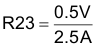Select R23 to be 0.2 Ω.

R22 and C15 form a pulse filter that helps provide additional immunity beyond the internal blanking time to the sudden voltage spike produced on R23 by the parasitic capacitance of the transformer and snubber network for Q4. The time constant for this filter is best determined experimentally but as a guideline should be no more than 25% of the minimum pulse width of the converter in actual operating conditions. Keeping the impedance low also helps with preventing noise coupling problems. For this converter 100 Ω and 150 pF were selected to give a time constant of 67 ns.

R21 is used to disable pulse skip mode if that is needed. To disable pulse skip mode, R21 must produce a 125 mVdc level or slightly higher at the CS pin. To calculate the required value:

Equation 23.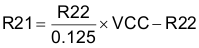Since VCC is 8 V:

Equation 24. R21 = 63 × R22

Select R21 to be 6.49 k to disable skip mode operation.

#### 8.2.2.6.1 Gate Drive Resistor

R16 limits the turn on and turn off speed of the power switch, Q4. The purpose for this is controlling the voltage spike at the drain of Q4 turn off. Selection of this resistor value should be done in conjunction with EMI compliance testing. Slowing the turn off time of Q4 will reduce EMI but also increase power dissipation in Q4. A general range of values to consider would be 0 Ω to 10 Ω for this converter. 4.7 Ω was chosen as the best overall solution for this converter.

#### 8.2.2.6.2 VCC Capacitor

C17 provides filtering for the internal linear regulator. Selection is somewhat arbitrary and was picked as 1 µF per recommendations above.

#### 8.2.2.6.3 Startup Circuit

The startup circuit for this converter illustrates a technique for starting the converter quickly without the need to wait for the larger VIN capacitance to be trickle charged through high impedance from the bulk voltage. It also allows the steady state impedance connected to the bulk voltage to be higher than otherwise possible, reducing power dissipation. The circuit consists of a series pass regulator (R1, R2, R5, Q1, D5 and C6) from the bulk voltage supply to VIN, a series pass regulator from the rectified AUX winding to VIN (C12, D8, Q3, R12 and D9) and a turn off circuit that turns off the bulk regulator once the converter is running (D7, R10 and Q2).

Q1 is selected for small size and the ability to withstand the maximum bulk voltage. A BSS127 is selected for its high maximum drain voltage of 600 V.

R2 is selected as 10k simply to limit current through Q1 to less than 50 mA per the BSS127 data sheet, at the maximum possible bulk voltage.

Equation 25.The voltage the bulk regulator supplies is equal to the zener voltage of D5 less the threshold voltage of Q1, typically 4 V. Since the controller requires a maximum of 23 V to start, the zener voltage must be at least 27 V. D5 is selected as a 27 V device, BZX585-C27.

The bulk series regulator is turned on by R1 and R5. These are only required to supply enough current to bias D5 and overcome any leakage in Q2, approximately 10 µA. To guarantee operation, bias the circuit with 25 µA minimum.

Equation 26.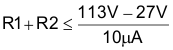The sum of R1 and R2 must be less than 8.6 MΩ. R1 and R2 are selected as 1.5 M each for common values.

C6 is simply a time delay to soften the startup of the bulk regulator and was arbitrarily chosen as 10 nF.

Turning to the AUX regulator, D8 protects the b-e junction of Q3 while the bulk regulator is active. It is a low current device that must withstand 27 V minimum. A TS4148 was chosen for this purpose.

Q3 is the pass element for the AUX regulator and is again low current. The only requirement is that the Vce rating be greater than the maximum rectified AUX voltage of 36 V. A common MMBT2222A was chose for this function.

The voltage supplied to the controller VIN pin by the AUX regulator is determined by voltage on C13 less 1.4 V for the drop across D8 and Q3 when the voltage on C13 is less than the zener voltage of D9 or the zener voltage of D9 less 1.4 V when the voltage on C13 is higher than the zener voltage of D9. The maximum voltage supplied to the controller is the zener voltage of D9 less 1.4 V. Picking a zener voltage of 15 V lets the controller run at approximately 13.6 V under normal conditions. A BZX585-C15 is selected.

R12 provides bias for D9 and base drive for Q3. Bias for D9 is small compared to the required base drive for Q3. The current required from the regulator is the sum of the controller operating current and the drive current for Q4. The drive current for Q4 depends on the operating frequency and the total gate charge of Q4 at 13.6 V.

Equation 27. IREG > IVIN + IDRV
Equation 28. IVIN = 3.5 mA
Equation 29. IDRV = 40 nC × 145 kHz = 5.8 mA

The regulator must supply a minimum of about 9.5 mA for the controller to function. From the MMBT2222A datasheet, the minimum current gain is 75 for 10-mA collector current. The worst case base drive occurs when the output is programmed for 8 V, giving 12 V available to the collector of Q3 and to the base drive resistor R12. To get a minimum of 10 mA from the regulator requires 133 µA of base drive current. The VIN voltage cannot fall below 7.25 V or the controller will shut down. R12 must satisfy the following relationship:

Equation 30.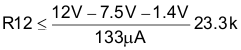R12 was picked as 22 k for this application.

The bulk regulator turn off circuit simply turns Q1 off when the voltage on C13 exceeds the zener voltage of D7 plus the b-e voltage of Q2 (0.7 V) and whatever voltage is required to get sufficient base drive through R10. The required collector current is at the maximum bulk voltage of 255 V.

Equation 31.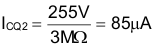Current gain for the selected MMBT2222A is over 100 at this level so the base drive required is only 8.5 µA. Picking R10 as 47 k requires only an additional 400 mV on C13 to effect turn off of the bulk regulator. The bulk regulator should turn off before the voltage on C13 reaches 12 V. Picking a zener voltage of 10 V with the BZX585-C10 ensures that the bulk regulator will turn off at no more than 11.8 V.

#### 8.2.3 Application Curves

All test results use 115-Vac input and 2200-µF external load capacitance.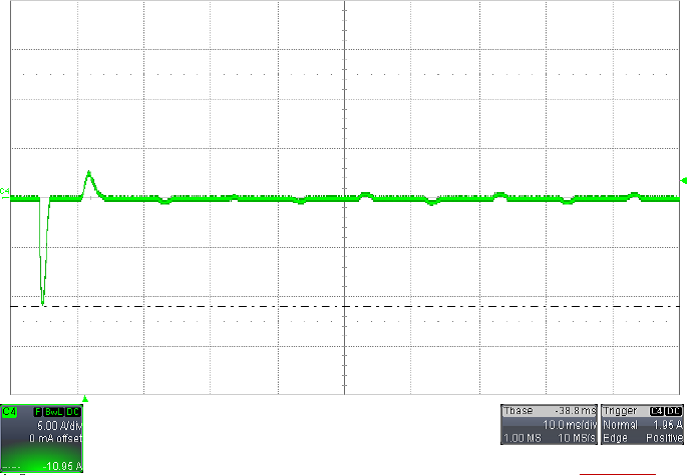Figure 15. AC Inrush Current, No Load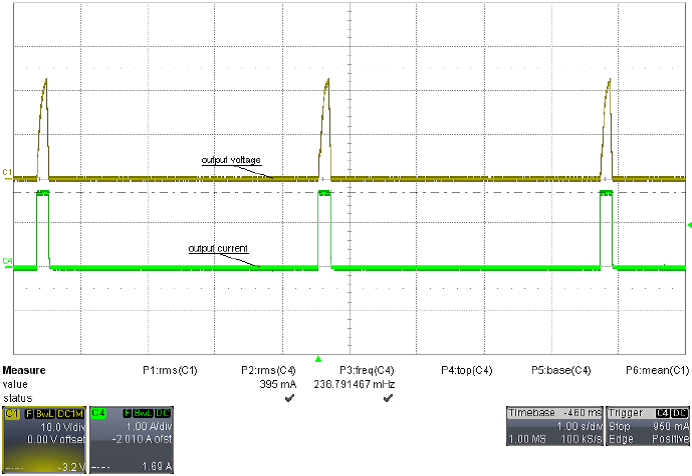Figure 17. Output Overload Hiccup Protection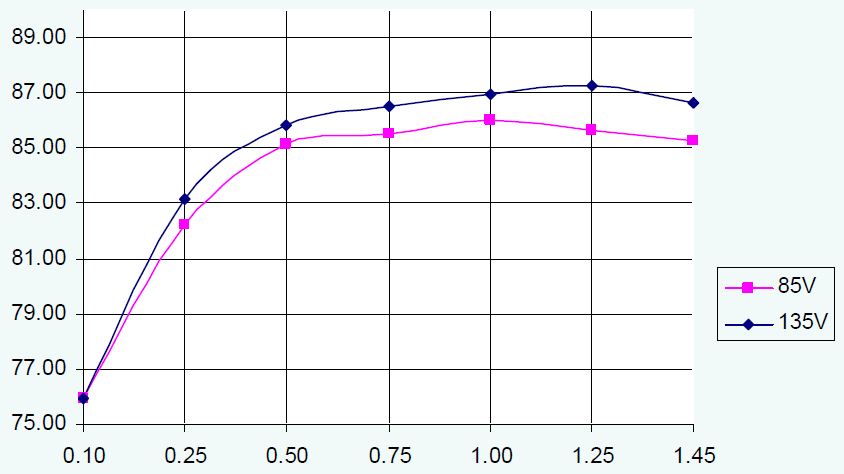Figure 19. Converter Efficiency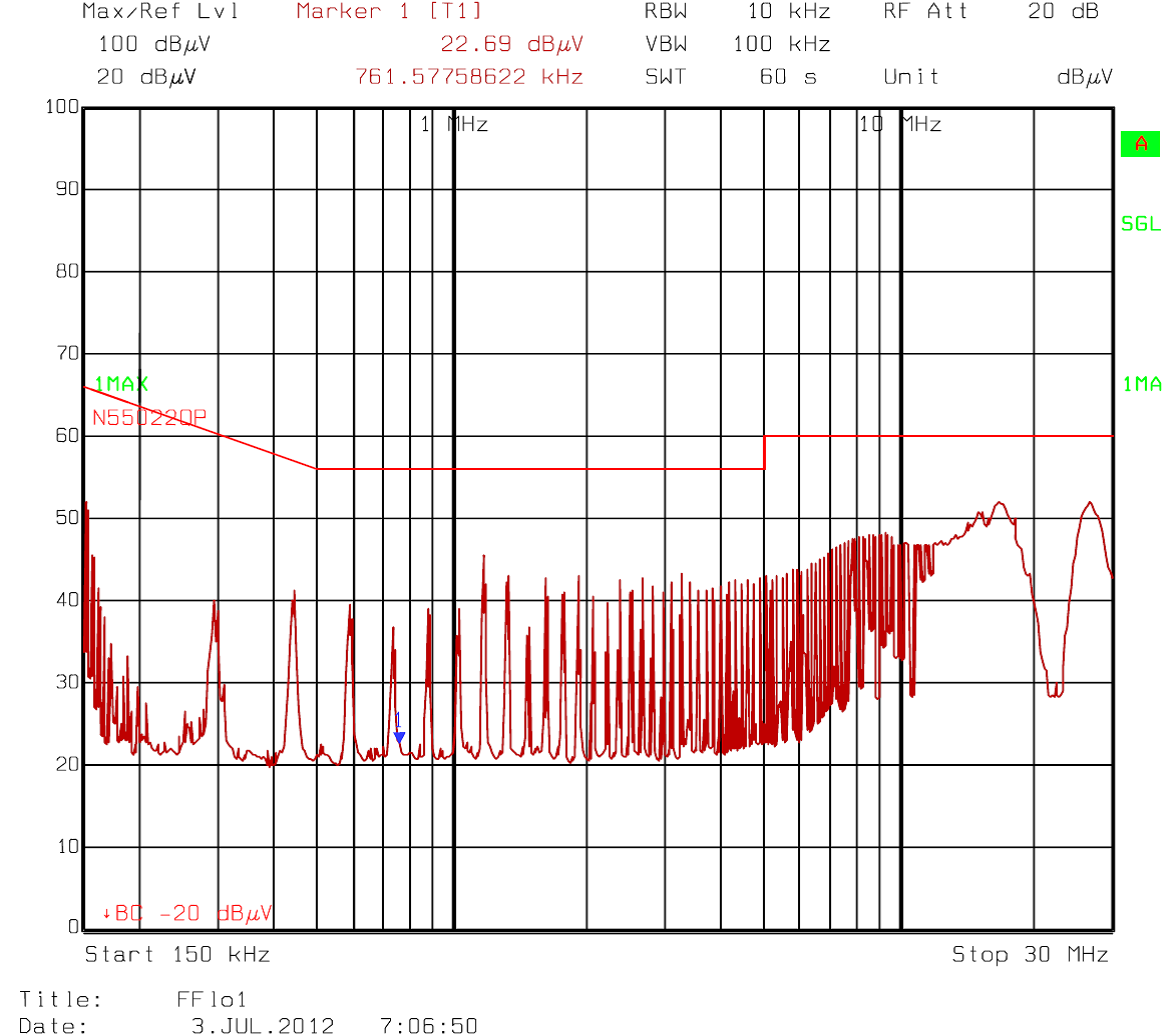Figure 21. Quasi-Peak EMI Measurement, Not Done in Certified Lab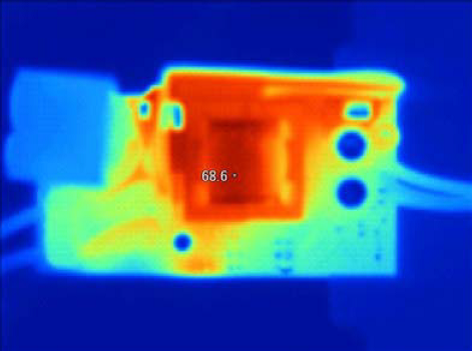Figure 23. Thermal Image, Top Side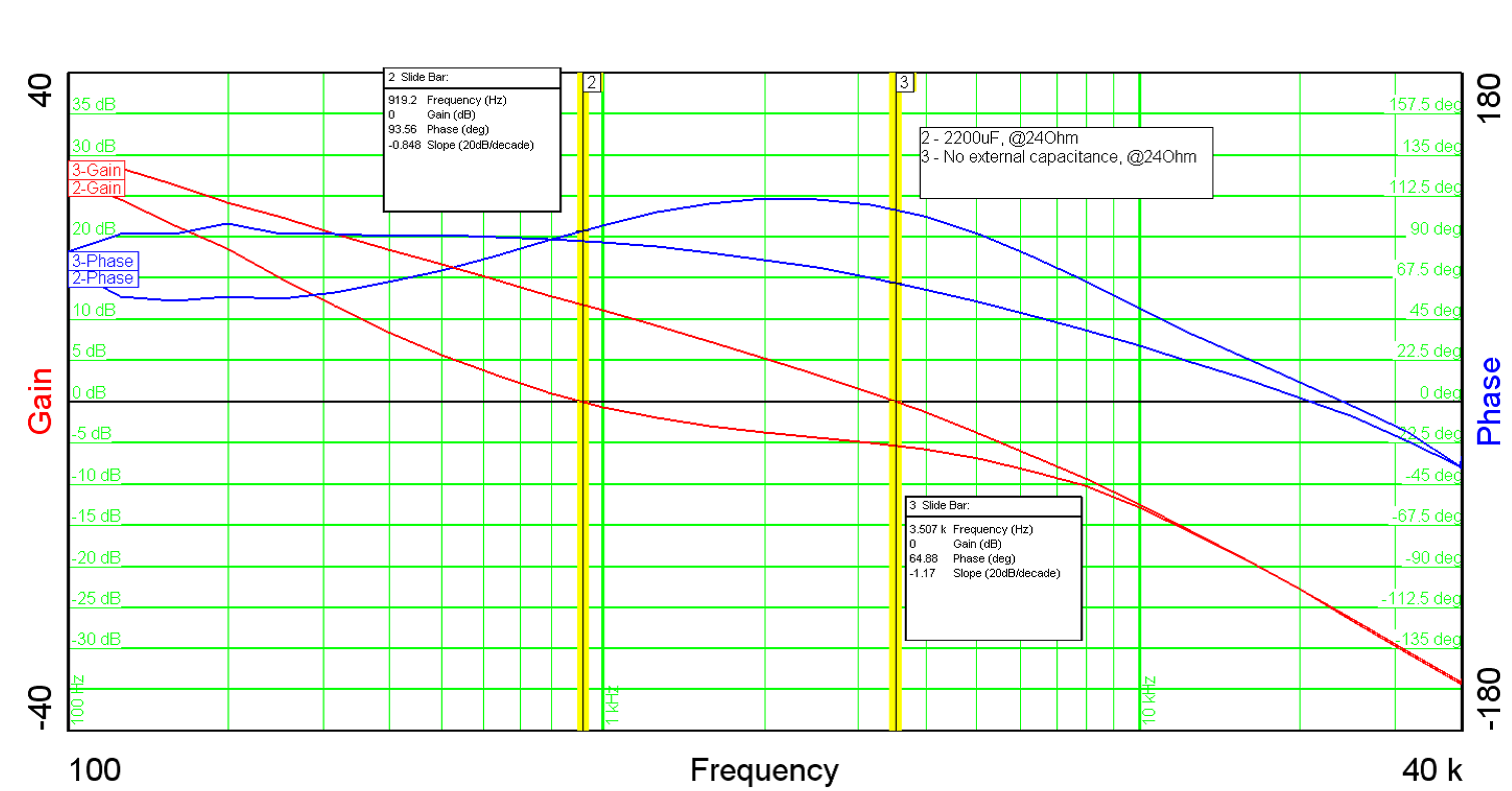Figure 25. Loop Response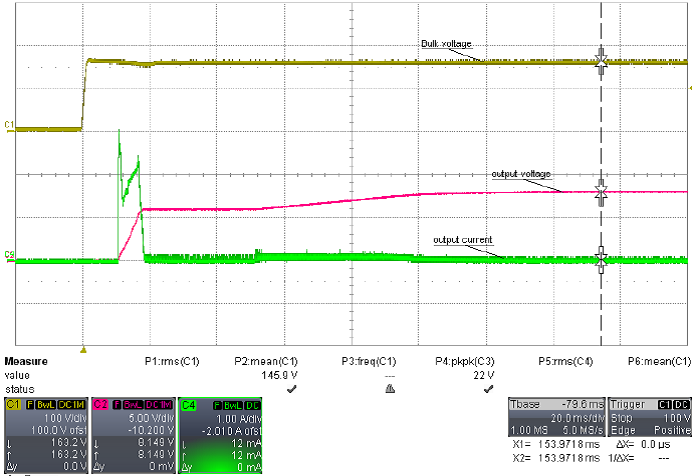Figure 16. Bulk Voltage, Output Voltage and Output Current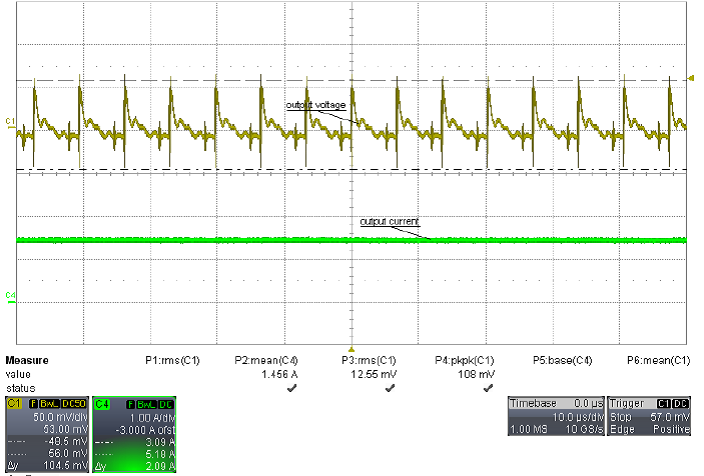Figure 18. Output Ripple: 108 mVpp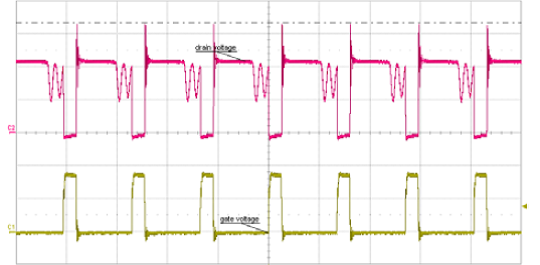Figure 20. Typical Switching Waveforms
Red: Q4 Drain Voltage, Yellow: Q4 Gate VoltageFigure 22. Average EMI measurement, Not Done in Certified Lab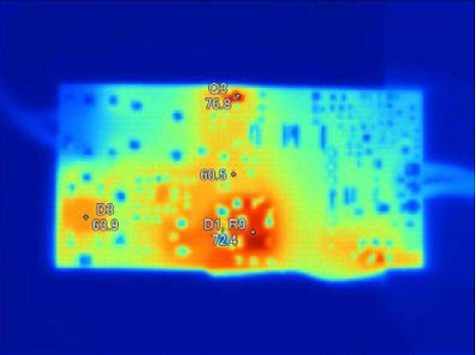Figure 24. Thermal Image, Bottom Side